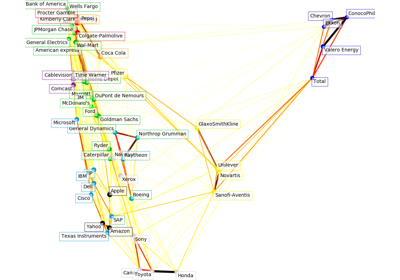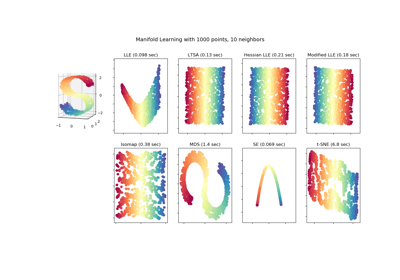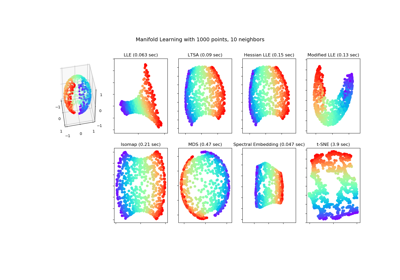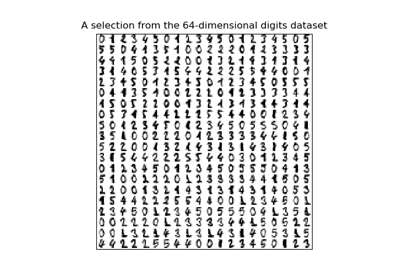# sklearn.manifold.LocallyLinearEmbedding¶

class sklearn.manifold.LocallyLinearEmbedding(n_neighbors=5, n_components=2, reg=0.001, eigen_solver='auto', tol=1e-06, max_iter=100, method='standard', hessian_tol=0.0001, modified_tol=1e-12, neighbors_algorithm='auto', random_state=None, n_jobs=None)[source]

Locally Linear Embedding

Read more in the User Guide.

Parameters
n_neighborsinteger

number of neighbors to consider for each point.

n_componentsinteger

number of coordinates for the manifold

regfloat

regularization constant, multiplies the trace of the local covariance matrix of the distances.

eigen_solverstring, {‘auto’, ‘arpack’, ‘dense’}

auto : algorithm will attempt to choose the best method for input data

arpackuse arnoldi iteration in shift-invert mode.

For this method, M may be a dense matrix, sparse matrix, or general linear operator. Warning: ARPACK can be unstable for some problems. It is best to try several random seeds in order to check results.

denseuse standard dense matrix operations for the eigenvalue

decomposition. For this method, M must be an array or matrix type. This method should be avoided for large problems.

tolfloat, optional

Tolerance for ‘arpack’ method Not used if eigen_solver==’dense’.

max_iterinteger

maximum number of iterations for the arpack solver. Not used if eigen_solver==’dense’.

methodstring (‘standard’, ‘hessian’, ‘modified’ or ‘ltsa’)
standarduse the standard locally linear embedding algorithm. see

reference 

hessianuse the Hessian eigenmap method. This method requires

n_neighbors > n_components * (1 + (n_components + 1) / 2 see reference 

modifieduse the modified locally linear embedding algorithm.

see reference 

ltsause local tangent space alignment algorithm

see reference 

hessian_tolfloat, optional

Tolerance for Hessian eigenmapping method. Only used if method == 'hessian'

modified_tolfloat, optional

Tolerance for modified LLE method. Only used if method == 'modified'

neighbors_algorithmstring [‘auto’|’brute’|’kd_tree’|’ball_tree’]

algorithm to use for nearest neighbors search, passed to neighbors.NearestNeighbors instance

random_stateint, RandomState instance or None, optional (default=None)

If int, random_state is the seed used by the random number generator; If RandomState instance, random_state is the random number generator; If None, the random number generator is the RandomState instance used by np.random. Used when eigen_solver == ‘arpack’.

n_jobsint or None, optional (default=None)

The number of parallel jobs to run. None means 1 unless in a joblib.parallel_backend context. -1 means using all processors. See Glossary for more details.

Attributes
embedding_array-like, shape [n_samples, n_components]

Stores the embedding vectors

reconstruction_error_float

Reconstruction error associated with embedding_

nbrs_NearestNeighbors object

Stores nearest neighbors instance, including BallTree or KDtree if applicable.

References

R62e36dd1b056-1

Roweis, S. & Saul, L. Nonlinear dimensionality reduction by locally linear embedding. Science 290:2323 (2000).

R62e36dd1b056-2

Donoho, D. & Grimes, C. Hessian eigenmaps: Locally linear embedding techniques for high-dimensional data. Proc Natl Acad Sci U S A. 100:5591 (2003).

R62e36dd1b056-3

Zhang, Z. & Wang, J. MLLE: Modified Locally Linear Embedding Using Multiple Weights. http://citeseerx.ist.psu.edu/viewdoc/summary?doi=10.1.1.70.382

R62e36dd1b056-4

Zhang, Z. & Zha, H. Principal manifolds and nonlinear dimensionality reduction via tangent space alignment. Journal of Shanghai Univ. 8:406 (2004)

Examples

>>> from sklearn.datasets import load_digits
>>> from sklearn.manifold import LocallyLinearEmbedding
>>> X.shape
(1797, 64)
>>> embedding = LocallyLinearEmbedding(n_components=2)
>>> X_transformed = embedding.fit_transform(X[:100])
>>> X_transformed.shape
(100, 2)


Methods

 fit(self, X[, y]) Compute the embedding vectors for data X fit_transform(self, X[, y]) Compute the embedding vectors for data X and transform X. get_params(self[, deep]) Get parameters for this estimator. set_params(self, \*\*params) Set the parameters of this estimator. transform(self, X) Transform new points into embedding space.
__init__(self, n_neighbors=5, n_components=2, reg=0.001, eigen_solver='auto', tol=1e-06, max_iter=100, method='standard', hessian_tol=0.0001, modified_tol=1e-12, neighbors_algorithm='auto', random_state=None, n_jobs=None)[source]

Initialize self. See help(type(self)) for accurate signature.

fit(self, X, y=None)[source]

Compute the embedding vectors for data X

Parameters
Xarray-like of shape [n_samples, n_features]

training set.

yIgnored
Returns
selfreturns an instance of self.
fit_transform(self, X, y=None)[source]

Compute the embedding vectors for data X and transform X.

Parameters
Xarray-like of shape [n_samples, n_features]

training set.

yIgnored
Returns
X_newarray-like, shape (n_samples, n_components)
get_params(self, deep=True)[source]

Get parameters for this estimator.

Parameters
deepbool, default=True

If True, will return the parameters for this estimator and contained subobjects that are estimators.

Returns
paramsmapping of string to any

Parameter names mapped to their values.

set_params(self, **params)[source]

Set the parameters of this estimator.

The method works on simple estimators as well as on nested objects (such as pipelines). The latter have parameters of the form <component>__<parameter> so that it’s possible to update each component of a nested object.

Parameters
**paramsdict

Estimator parameters.

Returns
selfobject

Estimator instance.

transform(self, X)[source]

Transform new points into embedding space.

Parameters
Xarray-like of shape (n_samples, n_features)
Returns
X_newarray, shape = [n_samples, n_components]

Notes

Because of scaling performed by this method, it is discouraged to use it together with methods that are not scale-invariant (like SVMs)

## Examples using sklearn.manifold.LocallyLinearEmbedding¶